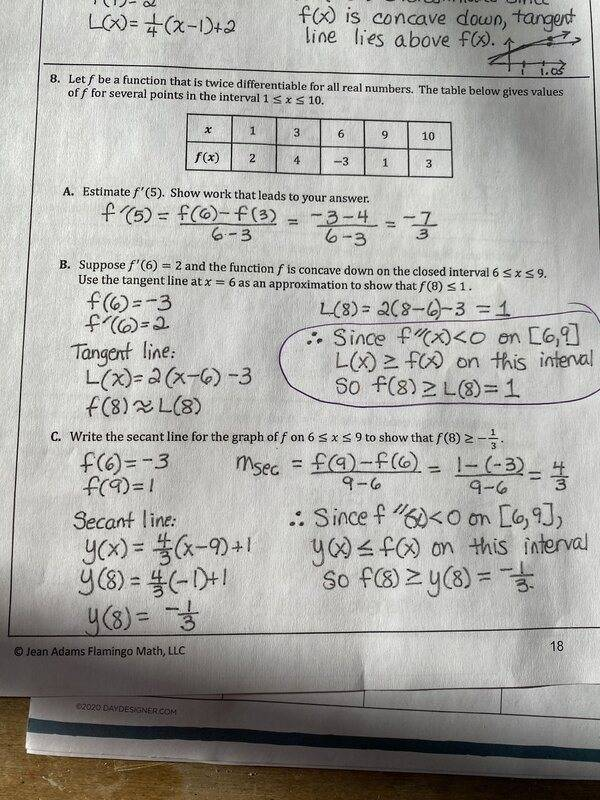# Concavity and Tangent Functions

Strand9202
Homework Statement:
The problem is below. I was asked to explain what is meant in the circled part.
Relevant Equations:
None
Here is the problem (8b). I was asked to write out why the circled part was true.I know that since the function is concave down then f"(x)<0. That is a fact. What I am having trouble with is why they can say the next part.

What I thought was L(x) is the tangent line and all tangent lines are above a concave down function, but not sure that is correct or true.

$$L(x)\ge f(x) \quad \Rightarrow\quad L(8) \ge f(8)\quad \Rightarrow \quad f(8)\le L(8) = 1$$q.e.d.